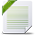# Decision Optimization

View Only

## Cplex error#### Anika TabassumWed July 01, 2020 04:02 AM#### Daniel JunglasWed July 01, 2020 04:05 AM#### Anika TabassumWed July 01, 2020 12:26 PM• #### 1.  Cplex error

Posted Wed July 01, 2020 04:02 AM
using CP;

// Set of inputs
int i=...; //set of origins
int j=...; //set of destinations
int t=...;//set of time step

range origins=1..i;
range destinations=1..j;
range time=1..t;

tuple demand {
int demand;
int origin;
int destination;
int time;
}

demand dm[origins][destinations][time]=...;

float p=...;

dvar int+ l[origins][destinations][time];
dvar int+ u[origins][destinations][time];

dexpr float cost1= sum(i in origins, j in destinations, t in time)(p*u[i][j][t]);

//Objective Function
minimize cost1;

subject to{
forall(i in origins, j in destinations, t in time)
u[i][j][t]== u[i][j][t-1] + dm[i][j][t]- l[i][j][t];
}

The error is Operator not available for dvar int+ + <demand:int,origin:int,destination:int,time:int>.

Data:
i=2;
j=2;
t=2;
p = 0.1;
dm = [ <30, 1, 1, 1>,
<80, 2, 1, 1> ];

What is the problem here?
Thank you.

------------------------------
Anika Tabassum
------------------------------

• #### 2.  RE: Cplex error

Posted Wed July 01, 2020 04:05 AM
In
`forall(i in origins, j in destinations, t in time)`
`    u[i][j][t]== u[i][j][t-1] + dm[i][j][t]- l[i][j][t];`
you are trying to add integer variable u with tuple dm. You probably mean something like dm[i][j][t].demand?

------------------------------
Daniel Junglas
------------------------------

• #### 3.  RE: Cplex error

Posted Wed July 01, 2020 12:26 PM
Edited by Anika Tabassum Wed July 01, 2020 12:51 PM
// Set of inputs
int i=...; //set of origins
int j=...; //set of destinations
int t=...;//set of time step

range origins=1..i;
range destinations=1..j;
range time=1..t;

tuple demandType {
int demands;
int origins;
int destinations;
int time;
}

demandType dm=...;//Demand of vehicles

float p=...;//Penalty cost

dvar int+ l[origins][destinations][time];//numbers of vehicles from origin i to destination j during t
dvar int+ u[origins][destinations][time];//unmet demand from origin i to destination j at the end of t

dexpr float cost1= sum(i in origins,j in destinations, t in time)(p*u[i][j][t]);

//Objective Function
minimize cost1;

subject to{
constraint1:
forall(i in origins, j in destinations, t in time:(t-1) in time)
u[i][j][t]== u[i][j][t-1] + dm.demands- l[i][j][t];

constraint2:
forall(i in origins, j in destinations, t in time)
l[i][j][t]<= dm.demands;

}
The model looks fine but the error is from the data file.
dm = { <19, 1,1,1>, <17, 2,1,1>, <18, 2,1,2>};
Data item "19" unexpected for "Demand[][destinations][time]".

------------------------------
Anika Tabassum
------------------------------

Attachment(s).oplproject   296 B 1 versionpartprac.mod   1011 B 1 version.project   270 B 1 versiondemand data.dat   281 B 1 version

• #### 4.  RE: Cplex error

Posted Wed July 01, 2020 01:19 PM
Hi

this looks the same question as https://stackoverflow.com/questions/62664474/how-do-i-solve-a-problem-with-tuples-in-cplex/62681376#62681376

you declared dm as

`demandType dm=...;//Demand of vehicles`

but dm is a tuple set so you d rather write

`{demandType} dm=...;//Demand of vehicles`

regards

------------------------------
ALEX FLEISCHER
------------------------------